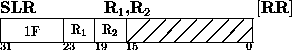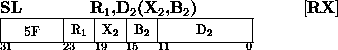Next: Test Under Mask Up: Insert Character Previous: Subtract halfword

### Subtract LogicalThe second operand is subtracted from the first operand, and the difference is placed in the first operand location. The occurrence of a carry out of the sign position is recorded in the condition code.

Logical subtraction is considered to be performed by adding the one's complement of the second operand and a low-order one to the first operand. All 32 bits of both operands participate, without further change to the resulting sign bit. The instruction differs from SUBTRACT in the meaning of the condition code and in the absence of the interruption for overflow.

If a carry out of the sign position occurs, the leftmost bit of the condition code is made one. In the absence of a carry, the bit is made zero. When the sum is zero, the rightmost bit of the condition code is made zero. For a nonzero sum, the bit is made one.

Resulting Condition Code:
0 ~ -
1 ~ Difference is not zero, with no carry
2 ~ Difference is zero, with carry
3 ~ Difference is not zero, with carry

Program Exceptions:
Access (fetch, operand 2 of SL only)

Programming Note
the use of the one's complement and the low-order one instead of the two's complement of the second operand results in the recognition of carry when subtracting zero or the maximum negative number. A zero difference cannot be obtained without a carry out of the sign position.Next: Test Under Mask Up: Insert Character Previous: Subtract halfword

A.Daviel,TRIUMF